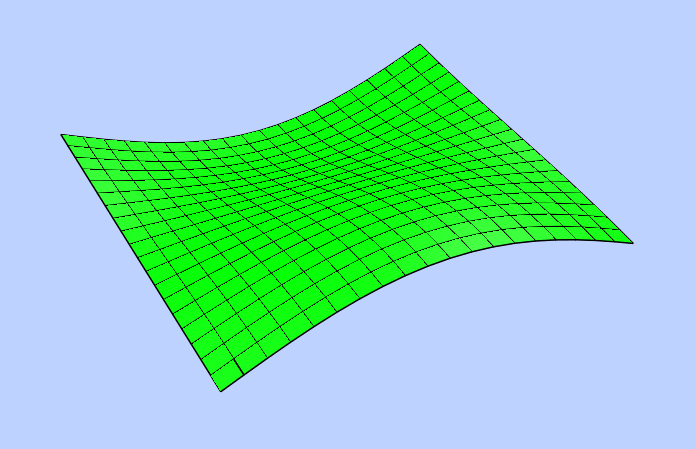# POLYMESH¶

Create a new m x n - polymesh entity, polymesh is a dxf-polyline entity!

`DXFEngine.``polymesh`(nrows, ncols, **kwargs)

Create a new polymesh entity.

nrows and ncols >=2 and <= 256, greater meshes have to be divided into smaller meshes.

The flags-bit POLYLINE_3D_POLYMESH is set.

Parameters: nrows (int) – count of vertices in m-direction, nrows >=2 and <= 256 ncols (int) – count of vertices in n-direction, ncols >=2 and <= 256

for kwargs see POLYLINE

## Methods¶

`Polymesh.``set_vertex`(row, col, point)

row and col are zero-based indices, point is a tuple (x,y,z)

`Polymesh.``set_mclosed`(status)
`Polymesh.``set_nclosed`(status)

Example:

```import math
from dxfwrite import DXFEngine as dxf

msize, nsize = (20, 20)
dwg = dxf.drawing('mesh.dxf')
mesh = dxf.polymesh(msize, nsize)
delta = math.pi / msize
for x in range(msize):
sinx = math.sin(float(x)*delta)
for y in range(nsize):
cosy = math.cos(float(y)*delta)
z = sinx * cosy * 3.0
mesh.set_vertex(x, y, (x, y, z))